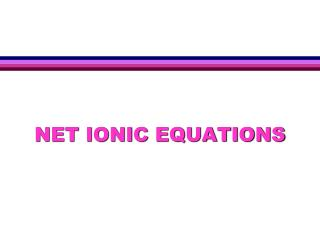DownloadDownload PresentationNET IONIC EQUATIONS

# NET IONIC EQUATIONS

Télécharger la présentation## NET IONIC EQUATIONS

- - - - - - - - - - - - - - - - - - - - - - - - - - - E N D - - - - - - - - - - - - - - - - - - - - - - - - - - -
##### Presentation Transcript

1. NET IONIC EQUATIONS

2. REMEMBER… Reactants (starting materials)  Products (ending materials) (g) = gas (l) = liquid (s) = solid (aq) = aqueous D = heat  = yields (dissolved in water)

3. CHEMICAL EQUATIONS There are three basic types of chemical equations: Molecular, Ionic, & Net ionic. • MOLECULAR EQUATIONS what we’re used to seeing HCl(aq) + NaOH(aq) NaCl(aq) + H2O (l)

4. CHEMICAL EQUATIONS • IONIC EQUATIONS have the substances which exist as ions written in ionic form. H+ (aq) + Cl- (aq) + Na+ (aq) + OH-(aq) Na+ (aq) + Cl- (aq) + H2O (l)

5. CHEMICAL EQUATIONS • NET IONIC EQUATIONS are ionic equations with the Spectator ions removed. H+ (aq) + OH-(aq) H2O (l) • SPECTATOR IONS do not participate in a reaction (that is they do not react to form a new substance).

6. TO WRITE COMPLETE IONIC EQUATION • Start with a balanced molecular equation. • Break all soluble strong electrolytes (compounds with (aq) beside them) into their ions • indicate the correct formula and charge of each ion • indicate the correct number of each ion • write (aq) after each ion • Bring down all compounds with (s), (l), or (g) unchanged.

7. TO WRITE COMPLETE IONIC EQUATION • 2 Na3PO4 (aq) + 3 CaCl2 (aq)  6 NaCl (aq) + Ca3(PO4)2 (s) • The complete ionic equation is: • 6 Na+ (aq) + 2 PO43- (aq) + 3 Ca2+ (aq) + 6 Cl- (aq)  6 Na+ (aq) + 6 Cl- (aq) + Ca3(PO4)2 (s) • Notice Ca3(PO4)2 (s) – because it is a solid, you do not break it down into ions

8. TO WRITE NET IONIC EQUATION • The complete ionic equation is: • 6 Na+ (aq) + 2 PO43- (aq) + 3 Ca2+ (aq) + 6 Cl- (aq)  6 Na+ (aq) + 6 Cl- (aq) + Ca3(PO4)2 (s) • Some of the ions on the reactant side and the product side are exactly the same • The sodium and chloride ions in this reaction are referred to as spectator ions – THEY DID NOT PARTICIPATE IN THE REACTION

9. TO WRITE NET IONIC EQUATION • Cross out the spectator ions that are present. • Write the "leftovers" as the net ionic equation. 6 Na+ (aq) + 2 PO43- (aq) + 3 Ca2+ (aq) + 6 Cl- (aq)6 Na+ (aq) + 6 Cl- (aq) + Ca3(PO4)2 (s) Net Ionic Equation: 2 PO43- (aq) + 3 Ca2+ (aq)  Ca3(PO4)2 (s)

10. PRACTICE PROBLEMSCHEMICAL EQUATIONS Write the ionic and net ionic equation for the following unbalanced molecular equations. 1. Na2SO4(aq) + BaCl2(aq)  BaSO4(s) + NaCl(aq) 2. Al(s) + H2SO4(aq)  Al2(SO4)3(aq) + H2(g) 3. NO2(g) + H2O(l) + O2(g)  HNO3(aq) 4. HCl(aq) + Fe2(SO4)3(aq)  FeCl3(aq) + H2SO4(aq) Remember, (aq) stands for ions dissolved in water

11. CHEMICAL EQUATIONSanswers 1. Na2SO4(aq) + BaCl2(aq)  BaSO4(s) + 2NaCl(aq) Ionic: 2Na+ + SO42- + Ba2+ + 2Cl-  BaSO4(s) + 2Na+ + 2Cl- Net ionic: SO42-(aq) + Ba2+(aq)  BaSO4(s) • 2Al(s) + H2SO4(aq)  Al2(SO4)3(aq) + H2(g) Ionic: 2Al(s) + 2H+ + SO42-  2Al3+ +SO42- + H2(g) Net ionic: Al(s) + H+(aq)  Al3+(aq) + H2(g)

12. CHEMICAL EQUATIONSanswers 3. NO2(g) + H2O(l) + O2(g)  HNO3(aq) NO2(g) + H2O(l) + O2(g)  H+(aq) + NO3-(aq) the ionic and net ionic equations are the same! 4. HCl(aq) + Fe2(SO4)3(aq)  FeCl3(aq) + H2SO4(aq) H+ + Cl- + Fe3+ + SO42-  Fe3+ +Cl- + H+ + SO42- N.R. No reaction occurs! All species are spectator ions.

13. GROUP STUDY PROBLEM # 16 Balance the following molecular equations then write both the ionic & net ionic equations: 1. __Na2SO4(aq) + __AlCl3(aq)  __Al2(SO4)3(aq) + __NaCl(aq) 2. __NaBr(aq) + __Cl2(g)  __ NaCl(aq) + __Br2(l) 3. __SbCl3(aq) + __Na2S(aq)  __Sb2S3(s) + __NaCl(aq) 4. __Mg(OH)2(aq) + __ H2SO4(aq) __ H2O(l) + __MgSO4(aq)